# Popular Mathematics Manipulatives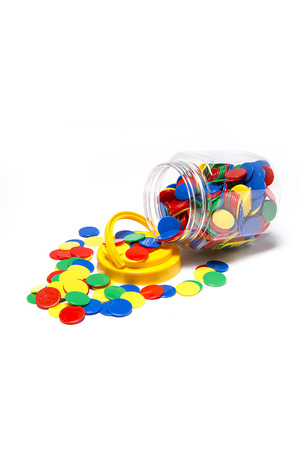Manipulatives provide students with a tactile and visual tool to help them learn maths. They make abstract mathematical concepts more concrete, increasing students’ understanding and ability to engage with lessons and solve problems. Here are some popular mathematics manipulatives to add to your classroom.

1. Dominoes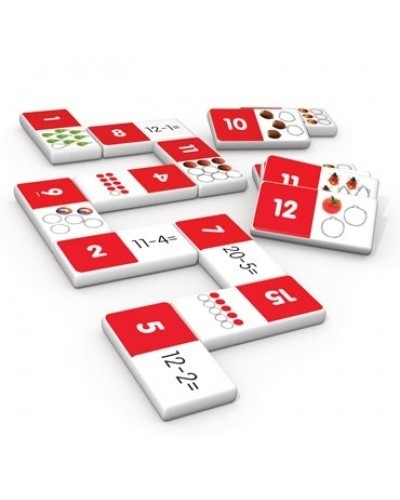These fun matching games will teach children the basic mathematics processes, such as addition, time, fractions, algebra, angles and more!

2. Counters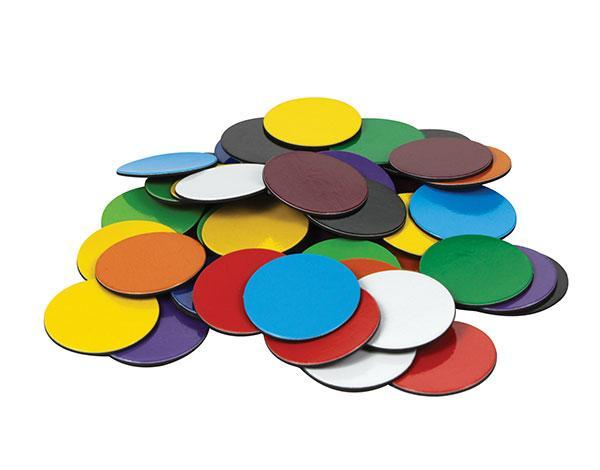Counters of all shapes, sizes and colours help students to understand and work out mathematics problems in a hands-on way.

3. Early Math 101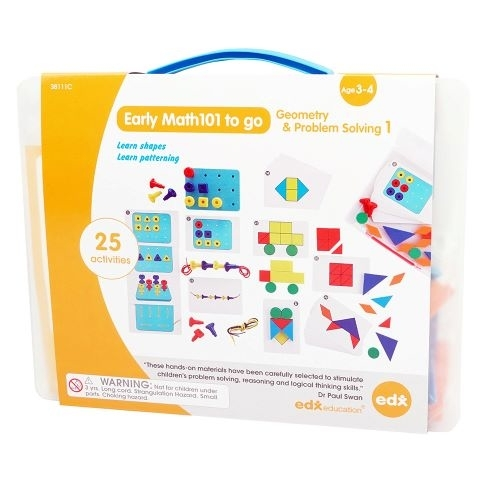These sets are the ideal resource to learn the names of shapes, patterning, problem solving skills, develop fine motor skills and hand-eye coordination.

4. Scales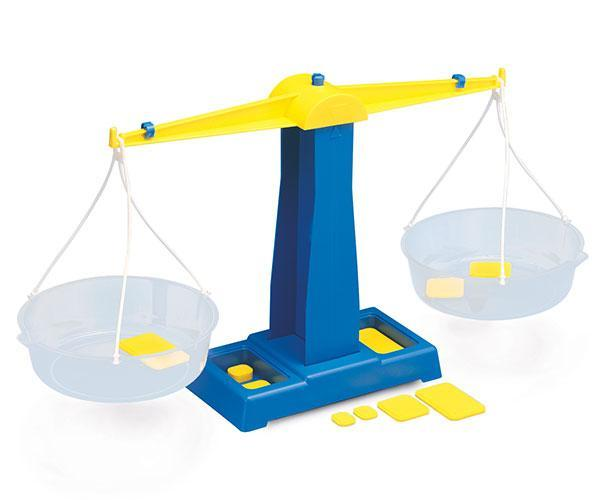These scales can be used to assist students in learning about volume and capacity, with both liquid and solid materials.

5. Base Ten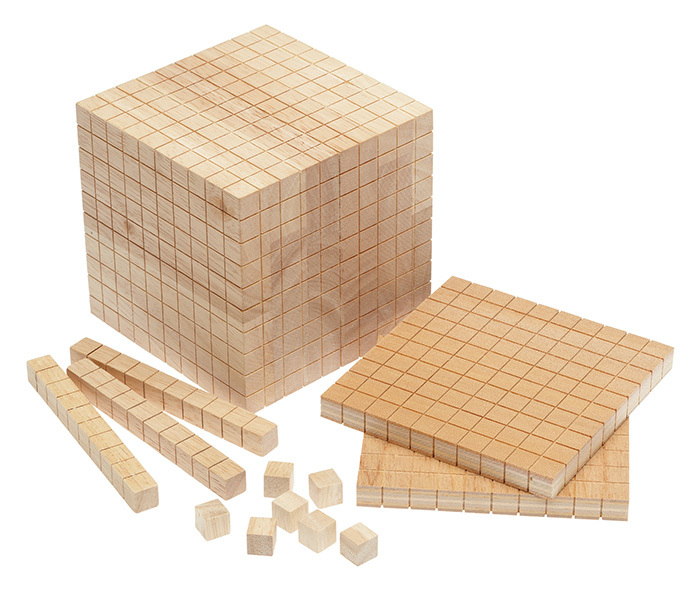Our range of base ten kits will help students understand the place value number system, and the relationship between units, tens, hundreds and thousands.